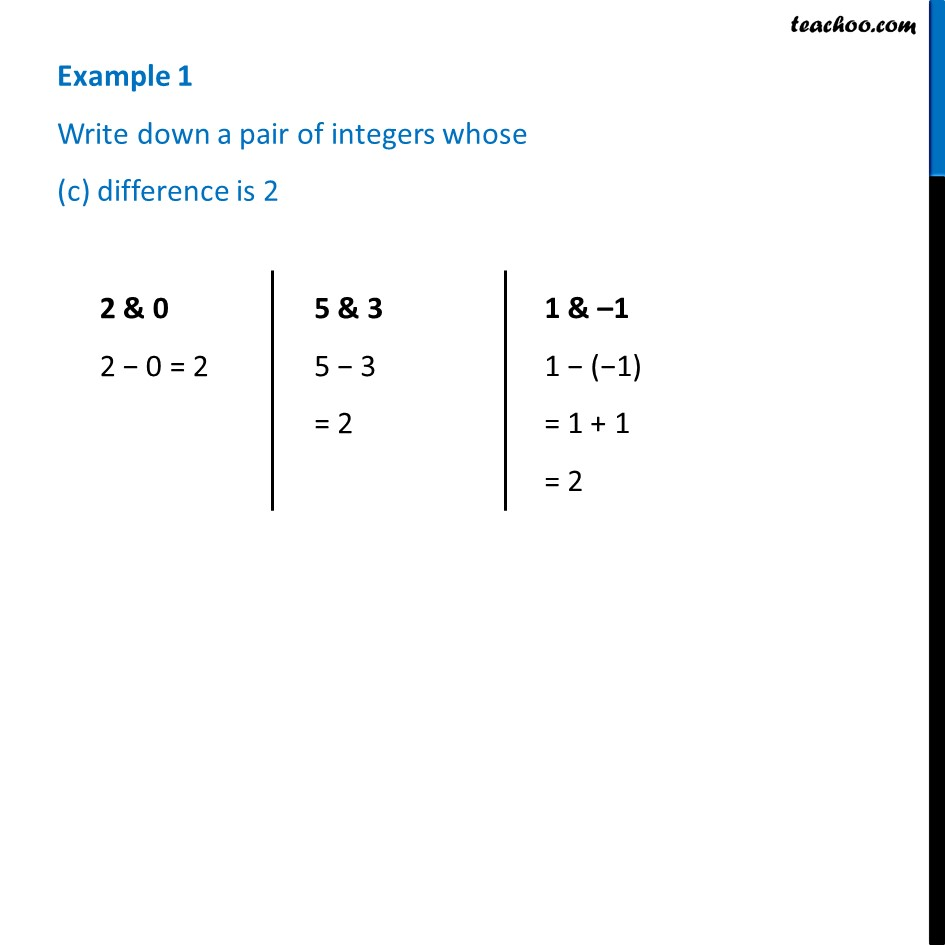Examples

Chapter 1 Class 7 Integers
Serial order wiseLearn in your speed, with individual attention - Teachoo Maths 1-on-1 Class

### Transcript

Example 1 Write down a pair of integers whose (c) difference is 2 2 & 0 2 − 0 = 2 5 & 3 5 − 3 = 2 1 & –1 1 − (−1) = 1 + 1 = 2 Example 1 Write down a pair of integers whose (d) sum is 0−2 & 2 −2 + 2 = 0 20 & −20 20 + (−20) = 20 − 20 = 0 9 & −9 = 9 + (−9) = 9 − 9 = 0